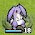## 2011年1月25日 星期二

### Rvalue Reference 與 String 的實作

Rvalue ReferenceC++0x 當中，一個非常重要的新功能。有了 Rvalue Reference，我們就可以容易地寫出具有 Moving Semantics 的 class。如果善用它，我們可以寫出更有效率的 C++ 程式碼。目前比較有名的編譯器如 GCC 或者 Visual C++ 都已經有 Rvalue Reference。

``````int a, b, c;
a = 1; b = 2; c = 3;  // (1)
a = b + c;  // (2)``````

``b+c = 0; // X``

``````string a("abc");
string b("def");
string c("ghi");

string all = a + b + c ＋ a;  // (3)``````

``````string operator+(string const &lhs, string const &rhs)  {
string result(lhs); // (4) copy lhs
result += rhs; // (5) concat rhs
return result;
}``````

1. 首先執行 a+b 的時候，我們要先複製一份 a（稱為 result1），再把 b 串接到 result1 的後面，最後回傳 result1。
2. 接著我們要計算 result1+c 的結果。我們必需先複製一份 result1（稱之為 result2），再把 c 串接到 result2 的後面，最後回傳 result2。
3. 最後我們要計算 result2+a 的結果，我們必需要先複製一份 result2（稱之為 result3），再把 a 串接到 result3 的後面，最後回傳 result3。

``````struct string_tmp {
string *ptr;
string_tmp(string *s) : ptr(s) { }
~string_tmp() { delete ptr; }
};

// Move Constructor
string::string(string_tmp rhs) {
internal_move(rhs);
}

// operator=
string &string::operator=(string_tmp rhs) {
internal_move(rhs);
return *this;
}

void string::internal_move(string_tmp with) {
if (buf) {
delete [] buf;
}

buf = with.ptr->buf;
buf_size = with.ptr->buf_size;
str_length = with.ptr->str_length;

with.ptr->buf = NULL;
with.ptr->buf_size = 0;
with.ptr->str_length = 0;
}

string_tmp operator+(string const &lhs, string const &rhs) {
string_tmp result(new string(lhs));
*(result.ptr) += rhs;
return result;
}

string_tmp operator+(string_tmp lhs, string const &rhs) {
*(lhs.ptr) += rhs;
return lhs;
}``````

Rvalue Reference 在 C++ 的 notation 如下：

``T &&rref = ... ;``

``string &&rref = a + b;``

``````string a;
string &&rref = a; // X``````

``string &&rref = std::move(a);``

``````// Move Constructor
string::string(string &&rhs)
: buf(NULL), buf_size(0), str_length(0) {

internal_move(rhs);
}

// operator=
string &string::operator=(string &&rhs) {
if (this == &rhs) {
return *this;
}
internal_move(rhs);
return *this;
}

void string::internal_move(string &with) throw () {
if (buf) {
delete [] buf;
}

buf = with.buf;
buf_size = with.buf_size;
str_length = with.str_length;

with.buf = nullptr;
with.buf_size = 0;
with.str_length = 0;
}

// operator+
string &&operator+(string &&lhs, string const &rhs) {
lhs += rhs;
return std::move(lhs); // convert lhs to r-value again
}``````

#### 4 則留言:

1.2.Lvalue 綁定到 Lvalue Reference

這行的Lvalue Reference是Rvalue reference的筆誤嗎？

3.＠Mr. Big Cat
對，我打錯了。應該要把該行的 Lvalue Reference 更正為 Rvalue Reference。謝謝指正。

4.作者已經移除這則留言。